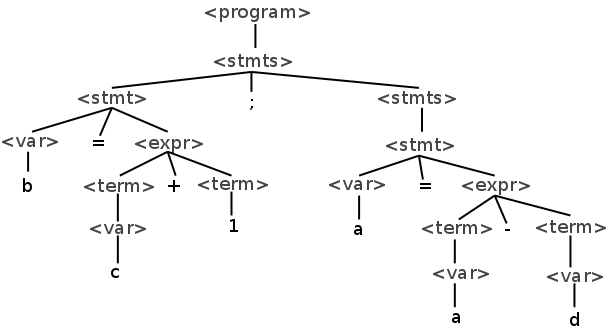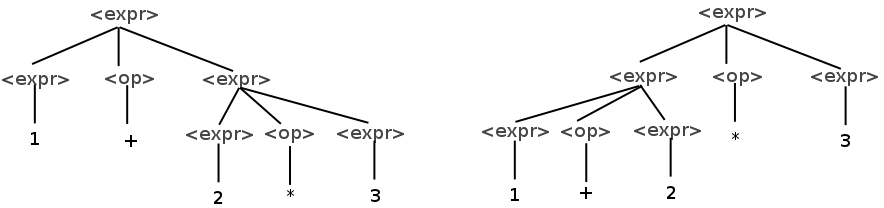# Syntax Analysis

## Overview

Syntax analysis, or parsing, is the problem of translating code in language into a parse tree using a given grammar.

 b = c + 1;a = a - d →Syntax analysis comes after lexical analysis. The input to the parser is a stream of tokens and the output is a parse tree.

The parser also catches syntax errors which occur when the input program does not conform to the rules of the grammar.

## Context-Free Grammars

Syntax is specified in the form of a context free grammar. CFGs contain a set grammar rules that describe the syntax of a language. CFGs only are able to describe a class of languages.

CFGs for languages are represented in Backus-Naur Form (BNF) which has a few elements:

• Nonterminals

Symbols that represent the syntactic structures in a language such as expressions and statements. They are typically contained in < and > symbols.

• Terminals

Lexemes in the language such as identifiers and other symbols.

• Rules

Rules have a left hand side which is a singe nonterminal and a right hand side which is a group of terminal and/or nonterminals.

• A start symbol

The symbol that defines how every overall program is formed.

## Example Grammar

Below is a very simple grammar for a small language.


<program> → <stmts>
<stmts> → <stmt> | <stmt> ; <stmts>
<stmt> → <var> = <expr>
<var> → a | b | c | d
<expr> → <term> + <term> | <term> - <term>
<term> → <var> | const


Convention has it that the first nonterminal, program, is the start symbol.

The | symbol means "or" and means that the nonterminal expands to one of the options on the right.

## Derivations

In a derivation, we start with the start symbol and repeatedly apply the rules of the grammar. Each time, we replace one nonterminal with the symbols on the right side of a rule with that nonterminal on the left hand side.

Below is an example derivation using the grammar above:


<program>
<stmts>
<stmt> ; <stmts>
<var> = <expr> ; <stmts>
b = <expr> ; <stmts>
b = <term> + <term> ; <stmts>
b = <var> + <term> ; <stmts>
b = c + <term> ; <stmts>
b = c + 1; <stmts>
b = c + 1; <stmt>
b = c + 1; <var> = <expr>
b = c + 1; a = <expr>
b = c + 1; a = <term> - <term>
b = c + 1; a = <var> - <term>
b = c + 1; a = a - <term>
b = c + 1; a = a - <var>
b = c + 1; a = a - d


Once there are no more nonterminals, the derivation is done. The result of derivation is a sentence in the language, or a set of lexemes that follows the syntax set forth in the grammar.

There are multiple ways to perform a derivation. The derivation above is a leftmost derivation because the leftmost symbol is the one that is replaced at each step.

We can also produce a rightmost derivation where we replace the rightmost symbol at each stage, or a derivation that switches between the two.

## Parse Trees

A parse tree is a visual representation of a derivation. Below is a parse tree of the example derivation above:The full sentence of nonterminals can be found by doing an in-order traversal of the parse tree.

The job of the parser is to construct the parse tree from a string in a language.

## Ambiguity

A grammar is ambiguous if a sentence in the grammar can have multiple distinct parse trees. Consider the following grammar for mathematical expressions:


<expr> → <expr> <op> <expr> | const
<op> → + | *


This grammar can be used to generate expressions like the following:


1 + 2 * 3


However this expression has two distinct parse trees:The fact that the precedence of operators is not enforced by the grammar gives this code two possible interpretations. On the left, multiplication is given the higher precedence, and on the right, addition is.

## Disambiguating Grammars

In order to avoid this, we can design the grammar to enforce the rule that the multiplication has higher precedence. The following grammar does this:


<expr> → <expr> + <term> | <term>
<term> → <term> * const | const


Here, the only possible parse tree has the multiplication done first.

## Parsing

Two types of parsers:

• Top-Down

Starts with the grammar's start symbol and follows the grammar rules as tokens come in. Easier to write by hand, but more restrictive.

• Bottom-Up

Matches tokens to terminals and replaces them with non-terminals according to the grammar. Hard to write by hand, but more powerful.

Parsing algorithms exist that can parse any grammar, but they are slow O(n^3). Compilers use algorithms that only work for a subset of grammars, but are linear.

## Top-Down "Recursive Descent" Parsing

In recursive descent parsing there is a function for each nonterminal in the grammar. That function is responsible for parsing strings that are matched to that nonterminal.

For the following grammar:


<expr> → <term> + <term>
| <term> - <term>

<term> → <factor> * <factor>
| <factor> / <factor>

<factor> → id | const | ( <expr> )


We can write a recursive descent parser as follows:


void expr() {
/* parse the first term */
term();

/* get the + or - */
if((token != PLUS) && (token != MINUS)) {
/* parse error! */
}

/* move on to the next token */
yylex();

/* parse the second term */
term();
}

This function matches the expr nonterminal. The convention is that each function leaves the next token in the "token" variable.

A real parser would also build the parse tree - or take some other action, but that is omitted here.

The term function would be similar:


void term() {
/* parse the first factor */
factor();

/* get the * or / */
if((token != TIMES) && (token != DIVIDE)) {
/* parse error! */
}

/* move on to the next token */
yylex();

/* parse the second factor */
factor();
}

Lastly, the factor could me parsed with this function:


void factor() {
/* check for the possibilities */
if(token == ID) {
yylex();

} else if(token == CONST) {
yylex();

} else if(token == LEFT_PARENS) {
yylex();

/* parse the expression */
expr();

/* check for the closing parens */
if(token == RIGHT_PARENS) {
yylex();
} else {
/* parse error! */
}
}
}

The sequence of function calls is essentially a traversal of the parse tree. As the parser runs, it can either construct a tree structure or generate code as it runs.

## LL Grammars

A recursive descent parser cannot be written for any grammar. One can be written for a class of grammar called LL.

The first L refers to the fact that the parser reads the input from the left. The second refers to the fact that the parser does a leftmost derivation.

There are a few constructs that an LL parser cannot handle. The first is if it must look more than one token ahead to determine which rule to take. If we have a grammar like this:


<if-stmt> → if ( <expr> ) <stmt>
| if ( <expr> ) <stmt> else <stmt>


The parser cannot know ahead of time which of the two rules it will follow. This can be solved with left factoring which transforms the rules into this:


<if-stmt> → if ( <expr> ) <stmt> <else-option>

<else-option> → else <stmt> | ε


This delays the decision until the parser has read the first portion of the if.

Another issue with LL grammars is that they do not support left-recursive rules like the following:


<stmts> → <stmts> ; <stmt> | ε


If a recursive descent parser tried to parse a rule like this, it would infinitely recurse. This must be translated into an equivalent right-recursive rule like so:


<stmts> → <stmt> ; <stmts> | ε


## Bottom Up Parsing

Bottom up parsing is a more common alternative to top-down parsing. Here, we start with the terminals and work our way up the tree. A bottom-up parser has two basic operations:

• Shift

Shifting reads another input symbol from the lexer. The symbol read in is a tree with one node.

• Reduce

Reducing joins existing trees as siblings of a larger tree.

During parsing, a bottom up parser maintains a parse stack holding terminals and nonterminals that have been parsed. At each step, the parser decides to either shift one token of input onto the stack, or to reduce tokens on the stack into a nonterminal using a grammar rule.

Consider the following grammar:


<stmt> → id = <term>

<term> → <term> + <factor>
| <factor>

<factor> → <factor> * <val>
| <val>

<val> → id | const


If we want to use this a bottom up parser to parse the code:


a = b + c * 4


Then the bottom up parser will perform the following actions:

 Step Stack Next Token Input Action 0 id = b + c * 4 shift 1 id = b + c * 4 shift 2 id = id + c * 4 shift 3 id = id + c * 4 reduce "val → id" 4 id = val + c * 4 reduce "factor → val" 5 id = factor + c * 4 reduce "term → factor" 6 id = term + c * 4 shift 7 id = term + id * 4 shift 8 id = term + id * 4 reduce "val → id" 9 id = term + val * 4 reduce "factor → val" 10 id = term + factor * 4 shift 11 id = term + factor * const shift 12 id = term + factor * const reduce "val → const" 13 id = term + factor * val reduce "factor → factor * val" 14 id = term + factor reduce "term → term + factor" 15 id = term reduce "stmt → id = term" 16 stmt Done!

## LR Grammars

The class of grammars parsable with a bottom up parser is called LR. The parser reads input from the left and produces a rightmost derivation.

For some grammars, there are times when the parser has two possible actions it can take. These are called conflicts.

A shift-reduce conflict is one where the parser could do a shift or reduce operation. Consider the following grammar:


<if-stmt> → if ( <expr> ) <stmt>
| if ( <expr> ) <stmt> else <stmt>


If the parser is in the following state:

 Stack Next Token Input Action if ( expr ) stmt else ... ???

Then the parser could shift or reduce. Clearly the parser in this situation should shift the else. This is called the dangling else problem and most parsers default to shifting in the presence of a shift-reduce conflict.

Reduce-reduce conflicts arise when the parser can reduce symbols on its stack to more than one nonterminal. The following grammar results in a reduce-reduce conflict:


<term> → id * id
<factor> → id * id


If the parser is in this state, it will could invoke either rule:

 Stack Next Token Input Action id * id ... ... ???

While not a problem for top-down parsers, grammar rules like this are not parsable by a bottom-up parser.

Bottom up parsers are constructed using parse tables. The table is indexed by the state we are currently in and the next input token. Constructing these tables by hand is very tedious.

There are tools that generate table-driven LR parsers from a context-free grammar. These are widely used to write compilers and interpreters.